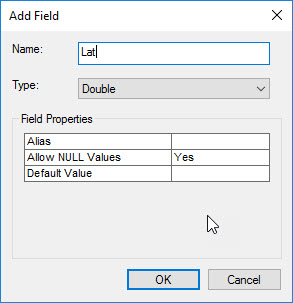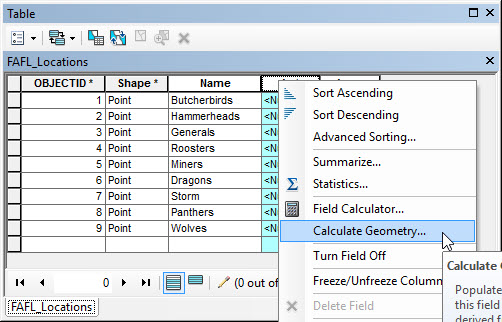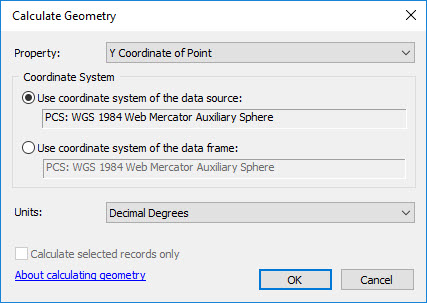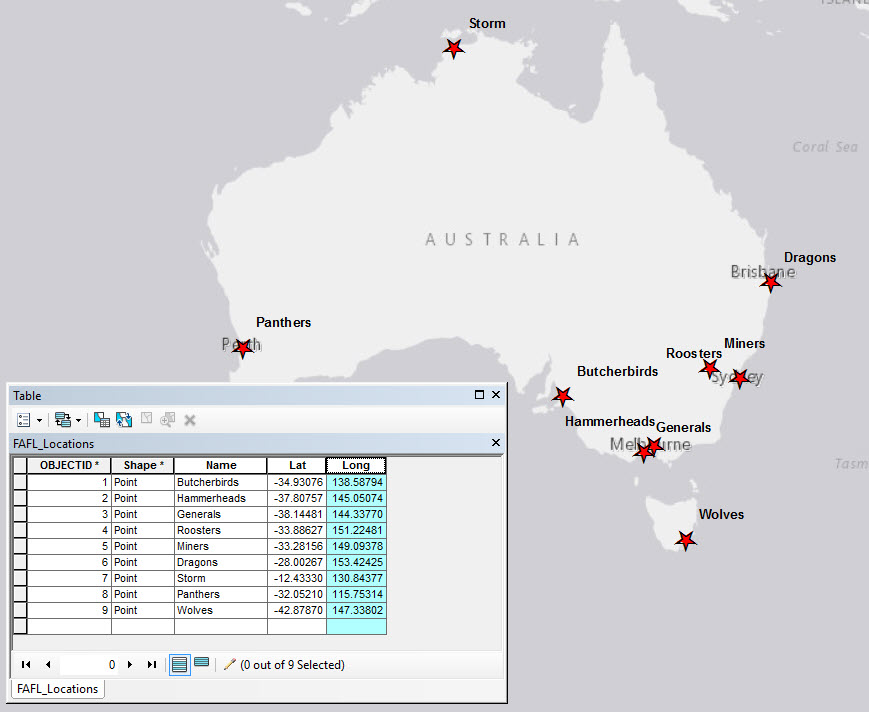English

# How To: Calculate latitude and longitude for point features

## Summary

Instructions provided describe how to use ArcMap to calculate the latitude and longitude for points in a point feature class.

## Procedure

In ArcGIS 10.1 and later versions, the Calculate Geometry tool can be used to provide the latitudes and longitudes of a point feature class.

In ArcGIS 9.x and 10.0, by using VBA Script Code in the Field Calculator, a field of type double can be populated with latitude or longitude values.

ArcGIS 10.1 and later versions:

1. Open the attribute table of the point feature class in ArcMap. Do not start an edit session.
2. Click the Table Options drop-down button, and select Add Field.1. In the Add Field window, name the field Lat (for latitude), with type Double. Click OK. Repeat this step and create another field named Long (for longitude).1. Start an edit session (Editor drop-down > Start Editing).1. Highlight the Lat field, right-click the header, and select Calculate Geometry.1. In the Calculate Geometry window, ensure that Property lists the Y Coordinate of Point, and to use the coordinate system of the data frame or feature layer. Set the Units as desired (in this scenario, Decimal Degrees). Click OK.1. Repeat steps 5 and 6, applying the X Coordinate of Point property to the Long field.
2. The Lat and Long fields are populated with latitudinal and longitudinal data.ArcGIS 9.x / 10.0:

1. Add a field of type Double to the attribute table to store either latitude or longitude values.
2. Start an edit session.
3. Open the attribute table.
4. Right-click the field and select Calculate Values.
5. Check the Advanced check box.
6. Paste the following code into the Pre-Logic VBA Code box:
```Dim Output As Double
Dim pPoint As IPoint
Set pPoint = [Shape]
Output = pPoint.X```
```Note:
The above code is for calculating longitude. X should be changed to Y to calculate latitude values.```
1. Type Output into the lower text box.
2. Click OK.